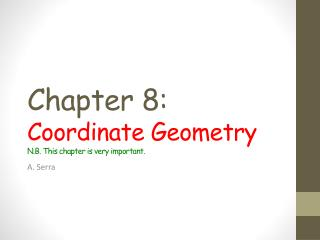Download PresentationChapter 8: C oordinate Geometry N.B. This chapter is very important.

# Chapter 8: C oordinate Geometry N.B. This chapter is very important.

Download Presentation## Chapter 8: C oordinate Geometry N.B. This chapter is very important.

- - - - - - - - - - - - - - - - - - - - - - - - - - - E N D - - - - - - - - - - - - - - - - - - - - - - - - - - -
##### Presentation Transcript

1. Chapter 8:Coordinate GeometryN.B. This chapter is very important. A. Serra

2. A. Distance between two points. Class work: Review: Number plane (2-D plane or Cartesian plane). x-axis, y-axis. Quadrants, coordinates. Distance between two points and the distance formula (page 254) Individual work: • Examples 1, 2, 3, 4: Do them & check your answers. • Exercise 8A1:1, Exercise 8A2:3,5 • Extra: Other exercises in 8A

3. B. Midpoints. CLASS WORK The midpoint formula (page 257) Example: exercise 10 on page 259. INDIVIDUAL WORK • Examples 5, 6, 7: Do them & check your answers. • Exercises 8B: 3(a,b,c), 7, 11. • Extra: Other exercises in 8B

4. C. Gradient.NB. Very important section. CLASS WORK The gradient of a line is a measure of its steepness and is equal to Vertical step/ horizontal step= rise/run= (y2-y1)/(x2-x1). Parallel lines have equal gradient, perpendicular negative reciprocals (-1/m). Exercise 8C.3: 6 (page 266) INDIVIDUAL WORK • Examples 8, 9, 10, 11, 12, 13 and 14: Do them & check your answers. • Exercises 8C1:1, 8C2: 2 (a,b,e), 8C3:2, 8C.4:1 • Extra: Other exercises in 8C.

5. D. Rates. CLASS WORK The gradient of a graph describes the RATE at which one quantity changes relative to the other. Eg, steepness, speed (d/t), … Exercise 8D4 (page 268) INDIVIDUAL WORK • Exercise 8D: 3 and 5 • Extra: Other exercises in 8D.

6. E. Vertical and horizontal lines. M.C. Escher – Relativity In the illustration three earth-planes cut across each other at right angles, and human beings are living on each of them. It is impossible for the inhabitants of different worlds to walk or sit or stand on the same floor, because they have different perceptions of what is horizontal and what is vertical. Yet they may well share the use of the same staircase. On the top staircase illustrated here, two people are moving side by side and in the same direction, and yet one of them is going downstairs and the other upstairs. Contact between them is out of the question because they live in different worlds and therefore can have no knowledge of each other's existence.

7. E. Vertical and horizontal lines. CLASS WORK All vertical lines have equations of the form x=constant, their gradient is undefined. All horizontal lines have equations of the form y=constant, their gradient is zero. Exercise 2 page 269 INDIVIDUAL WORK • Exercises 8E: 1, 3 • Extra: 8E (even numbers)

8. F. Equations of lines CLASS WORK The equation of a line is an equation which connects the x and y values for every point on the line. A point lies on a line if its coordinates satisfy its equation. m= (y-y1)/(x/x1) ; Y=mx+c (gradient-intercept) ; ax+by+c=0 (general equation) INDIVIDUAL WORK • Examples 15, 16, 17, 18, 19, 20, 21: Do them & check your answers. • Exercises 8F1 (a only) : 1, 3, 5, 7. • Exercises 8F2(a only) : 1 • Exercises 8F3(a only) : 1,3 • Extra: 8F (even numbers).

9. G. Graphing lines CLASS WORK Graphing lines by finding the intercepts. Remember: The x-intercept is found by letting y=0 The y-intercept is found by letting x=0 INDIVIDUAL WORK • Examples 22, 23, 24: Do them & check your answers. • Exercise 8G1: 1(a, b, c only). • Exercise 8G2: 1(a, b, c only) • Exercise 8G3: 3 • Extra: 8G (even numbers).

10. H. Perpendicular bisectors CLASS WORK The perpendicular bisector of AB is the set of all points which are the same distance from A and B. Exercise 8H2. Page 281. INDIVIDUAL WORK • Example 25: Do it & check your answers. • Exercise 8H: 1a, 3, 5 • Extra: 8G (even numbers).

11. Review • INDIVIDUAL WORK • Review Set 8A. (Do & check answers) • Extra: Review Set 8B, 8C and 8D • NEXT • …